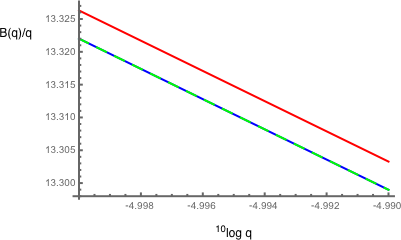# Asymptotics of a special function

In my research, I came up with a special function which I denote by $B(q)$ and is defined by the integral $$B(q)\equiv \int_{-\pi/2}^{\pi/2} \frac{\sin\left(\frac{q}{2}\tan\theta\right)}{\sin\theta}d\theta\ .$$ I only care about real $q$'s, but that is probably not important for my question. Using a simple change of variables $w=\frac{q}{2}\tan\theta$ this can also be written as $$B(q)=\int_{-\infty}^{\infty} \frac{\sin\left(w\right)}{w}\frac{1}{\sqrt{1+4 w^2/q^2}}dw$$ (if you're interested, this came up as one of the components of the response function of an elastic half-space).

I am interested in the asymptotics of $B(q)$. For large $q$ it is easy to show that $B(q)$ approaches $\pi$. My problem is with the small $q$ limit, $q\to0$. Numerically I have found that the leading order seems to behave as $B(q)=(\pi \gamma-\log(q))q+\mathcal{O}(q^2)$, where $\gamma$ is the Euler-Mascharoni constant. You can see in these plots that this is a very good guess (blue line is the approximation, red points are calculated numerically):What I need is a way to prove that this is indeed the asymptotic behavior of $B$. Any suggestions (or references to relevant techniques) will be much appreciated.

• Maple evaluates as (1/4)*q*MeijerG([[1/2], []], [[0, 0], [-1/2]], (1/16)*q^2)'which is not of much help, except that Maple can evaluate it quickly. Maple matched the graphs you show. Feb 17, 2016 at 17:07

Gradshteyn & Ryzhik equation 3.773.1 gives (for $$q>0$$)

$$\frac{1}{q}B(q)=\int_0^\infty \frac{\sin x}{x(q^2/4+x^2)^{1/2}}\,dx=\frac{1}{q}G^{21}_{13}\left(\frac{q^2}{16}\biggl|^1_{1/2,1/2,0}\right)$$ $$\qquad\qquad=1-\gamma+\log 4-\log q+{\cal O}(q^2)=1.80908-\log q+{\cal O}(q^2).$$

The asymptotic result desired by the OP is $$B(q)/q=\pi\gamma-\log q=1.81338-\log q$$. This is sufficiently close to the above answer that I am tempted to conclude that the correct order 1 term is actually $$1-\gamma+\log 4$$ instead of the conjectured $$\pi\gamma$$.

In support of this, the plot shows the numerical evaluation of the integral (green line) and the two asymptotes (blue and red lines). The upper red line is the asymptote guessed by the OP and the lower blue line is the asymptote obtained here, which seems to be the correct one.and this is j.c. 's plot, showing the difference between $$B(q)$$ and the two asymptotes (gold from the OP, and blue from this answer):• Also, replacing $w$ by $wq$ in the integral gives an integral of a dilate of "sinc" against $1/\sqrt{1+w^2}$, and (up to some normalizations...) those sinc dilates approach Dirac delta in a reasonable topology. This doesn't give lower-order terms, though, without more effort. Feb 17, 2016 at 15:51
• @Paul Garrett: This argument will yield the limit $q\to\infty$, not $q\to 0$. Feb 17, 2016 at 16:44
• Here are the differences (1) $B(q)-(1-\gamma+\log 4-\log q)q$ (in blue) and (2) $B(q)-(\pi\gamma-\log q)q$ (in gold) plotted in Mathematica imgur.com/TFx7tAL . To my eye it does seem that the blue curve has 0 slope at $q=0$, consistent with $O(q^2)$ corrections, whereas the gold curve has negative slope there, meaning that corrections to it do not seem to be $O(q^2)$.
– j.c.
Feb 17, 2016 at 18:43
• @j.c. --- thank you, I was just inserting a plot with a similar conclusion. Feb 17, 2016 at 18:56
• No problem, feel free to use my plot in your answer if you wish.
– j.c.
Feb 17, 2016 at 19:51